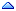Home » Other » General » Puzzle n°12 - Arithmetic expressions for a given result (24 game) *
Puzzle n°12 - Arithmetic expressions for a given result (24 game) * Wed, 01 June 2011 02:24Michel Cadot Messages: 68455Registered: March 2007 Location: Nanterre, France, http://... Senior MemberAccount Moderator
Here's a puzzle posted in OTN forum and named 24 game.

Quote:
My son just got a game called 24 each card has 4 numbers on it.
Here are the rules:
```object is to make 24 with all four numbers on the card.
you can add, subtract, multiply, and divide
you must use all four numbers, but use each only once.
you may use the result of an operation but only once
for example for the numbers 5 8 4 and 7  4 + 8 = 12   7 - 5 = 2   2 x 12 = 24```

And here's the test case:
```drop table game;
create table game (num integer);
insert into game values (5);
insert into game values (8);
insert into game values (4);
insert into game values (7);
commit;```

The result should be all expressions using the basic arithmetic operations and giving the result 24 using all given numbers once and only once.
The result for the example given above is: (4+8)*(7-5)

You can use any Oracle version you want but indicate it.

(Note: I found 156 different expressions.)

Good luck.
Michel

[Updated on: Wed, 01 June 2011 02:31]

Report message to a moderator

Re: Puzzle n°12 - Arithmetic expressions for a given result (24 game) * [message #517321 is a reply to message #509798] Mon, 25 July 2011 06:54Michel Cadot Messages: 68455Registered: March 2007 Location: Nanterre, France, http://... Senior MemberAccount Moderator
It seems nobody is interesting in this problem.

Here are 2 solutions (from 10g):
```with
numbers as ( -- All permutations of the given numbers
select replace(sys_connect_by_path(num, '#'),'#') v
from game
where connect_by_isleaf = 1
connect by nocycle prior num != num
),
oper as ( -- Allowed operators
select decode(level, 1,'-', 2,'+', 3, '*', 4,'/') op
from dual
connect by level <= 4
),
opers as ( -- All combinations of these operators
select a.op||b.op||c.op op
from oper a, oper b, oper c
),
-- All possible combinations of parentheses (numbered from 1 to 5)
parent as ( select level par from dual connect by level <= 5 ),
formulas as ( -- Build all possible formulas, assuming numbers have one digit
select decode (par,
-- case "((a.b).c).d"
1, '(('||substr(v,1,1)||substr(op,1,1)||substr(v,2,1)||')'||
substr(op,2,1)||substr(v,3,1)||')'||substr(op,3,1)||substr(v,4,1),
-- case "(a.(b.c)).d"
2, '('||substr(v,1,1)||substr(op,1,1)||'('||substr(v,2,1)||substr(op,2,1)||
substr(v,3,1)||'))'||substr(op,3,1)||substr(v,4,1),
-- case "(a.b).(c.d)"
3, '('||substr(v,1,1)||substr(op,1,1)||substr(v,2,1)||')'||
substr(op,2,1)||'('||substr(v,3,1)||substr(op,3,1)||substr(v,4,1)||')',
-- case "a.((b.c).d)"
4, substr(v,1,1)||substr(op,1,1)||'(('||substr(v,2,1)||substr(op,2,1)||
substr(v,3,1)||')'||substr(op,3,1)||substr(v,4,1)||')',
-- case "a.(b.(c.d))"
5, substr(v,1,1)||substr(op,1,1)||'('||substr(v,2,1)||substr(op,2,1)||
'('||substr(v,3,1)||substr(op,3,1)||substr(v,4,1)||'))')
formula
from numbers, opers, parent
)
select formula
from formulas
-- Restrict the formulas to those that results to 24
where 24 =
to_number(extractvalue(dbms_xmlgen.getXMLtype('select '||formula||' v from dual'),'//V'))
/```

```with
numbers as ( -- All permutations of the given numbers
select sys_connect_by_path(num, '#')||'#' v
from game
where connect_by_isleaf = 1
connect by nocycle prior num != num
),
oper as ( -- Allowed operators
select decode(level, 1,'-', 2,'+', 3, '*', 4,'/') op
from dual
connect by level <= 4
),
-- All possible combinations of parentheses (numbered from 1 to 5)
parent as ( select level par from dual connect by level <= 5 ),
formulas as ( -- Build all possible formulas, no assumption
select decode (par,
-- case "((a.b).c).d"
1, regexp_replace (
regexp_replace (
regexp_replace (
regexp_replace (
regexp_replace (v, '#', '((', 1, 1),
'#', op1.op, 1, 1),
'#', ')'||op2.op, 1, 1),
'#', ')'||op3.op, 1, 1),
'#', '', 1, 1),
-- case "(a.(b.c)).d"
2, regexp_replace (
regexp_replace (
regexp_replace (
regexp_replace (
regexp_replace (v, '#', '(', 1, 1),
'#', op1.op||'(', 1, 1),
'#', op2.op, 1, 1),
'#', '))'||op3.op, 1, 1),
'#', '', 1, 1),
-- case "(a.b).(c.d)"
3, regexp_replace (
regexp_replace (
regexp_replace (
regexp_replace (
regexp_replace (v, '#', '(', 1, 1),
'#', op1.op, 1, 1),
'#', ')'||op2.op||'(', 1, 1),
'#', op3.op, 1, 1),
'#', ')', 1, 1),
-- case "a.((b.c).d)"
4, regexp_replace (
regexp_replace (
regexp_replace (
regexp_replace (
regexp_replace(v, '#', '', 1, 1),
'#', op1.op||'((', 1, 1),
'#', op2.op, 1, 1),
'#', ')'||op3.op, 1, 1),
'#', ')', 1, 1),
-- case "a.(b.(c.d))"
5, regexp_replace (
regexp_replace (
regexp_replace (
regexp_replace (
regexp_replace (v, '#', '', 1, 1),
'#', op1.op||'(', 1, 1),
'#', op2.op||'(', 1, 1),
'#', op3.op, 1, 1),
'#', '))', 1, 1))
formula
from numbers, oper op1, oper op2, oper op3, parent
)
select formula
from formulas
-- Restrict the formulas to those that results to 24
where 24 =
to_number(extractvalue(dbms_xmlgen.getXMLtype('select '||formula||' v from dual'),'//V'))
/```

(In 11g you can use xmlquery instead of this complex formula with dbms_xmlgen.)

These solutions:
1/ Work on rational numbers, that is some intermediate results are not integers
2/ Display obvious equivalent solutions like (a+b)+(c+d)=a+(b+(c+d))=((a+b)+c)+d...

Next steps:
1/ Eliminate solutions with non integer intermediate results
2/ Eliminate obvious equivalent solutions

Good luck
Michel
 Previous Topic: please tell me Next Topic: Puzzle n°13 - light bulbs *
Goto Forum:

Current Time: Sat Jun 10 16:45:35 CDT 2023